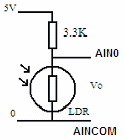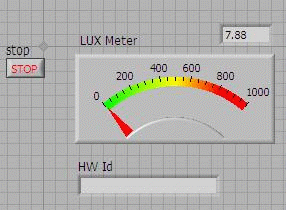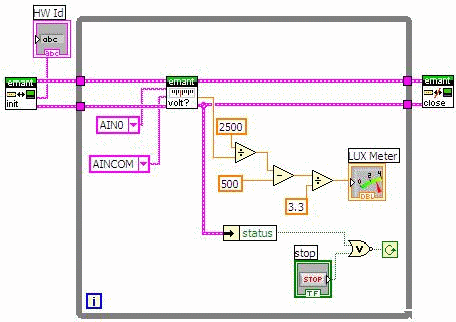# Measure Light Intensity using Light Dependent Resistor (LDR)The resistance of the Light Dependent Resistor (LDR) varies according to the amount of light that falls on it. The relationship between the resistance RL and light intensity Lux for a typical LDR is

\begin{equation} R_{L} = {500 \over Lux} \end{equation}

If the LDR connected to 5V through a 3.3K resistor, using the voltage divider rule, the output voltage of the LDR is

\begin{equation} V_{o} = {5 \times {R_{L} \over {R_{L} + 3.3}}} \end{equation}

Substituting RL from equation 1 into equation 2, we obtain the light intensity

\begin{equation} Lux = {{{2500 \over V_{o}} - 500} \over 3.3} \end{equation}

For a low cost LDR, at the same light intensity, the part to part variation in resistance can be as high as 50%. Therefore such a low cost LDR is seldom used for measuring light intensity but more for light presence/absence detection.

ParameterMinTypMaxUnitTest Cond
RL Light Resistance2010010 lux
5100 lux

Table 1: Typical Low Cost LDR Specification

If a more accurate light intensity measurement is required, we recommend using the Photodiode (see Application Note on Measuring Light Intensity using Photodiode)

The LabVIEW VI that reads the voltage across the LDR and converts the voltage to lux is called EMANT300 Example LDR.VI

## Front Panel## Block Diagram